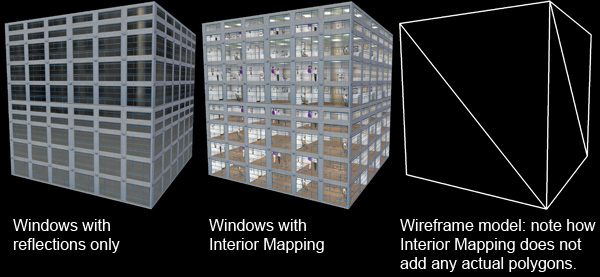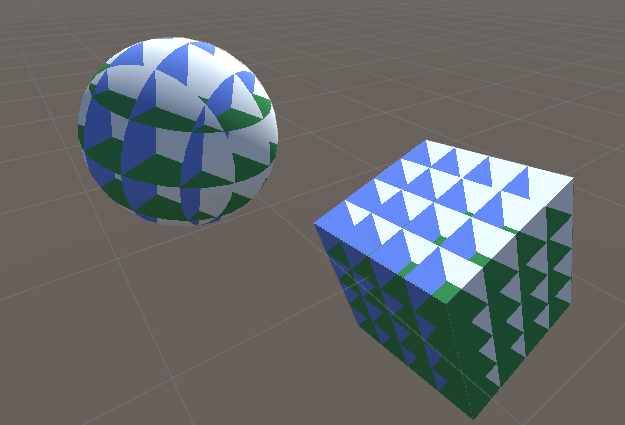## 2. Interior mapping

What if you have a city with tall buildings. To make the city more realistic you want the player to be able to see the interior of the buildings, so you have to model the interior of each single floor. But what if there's a better way? A better way is interior mapping, which is used in games like SimCity. This is the basic idea:Interior mapping is a shader technique used to simulate floors in buildings, and it was first proposed in this report: Interior mapping - A new technique for rendering realistic buildings. The very basic idea is this:

• Use planes to simulate the walls
• Fire a ray from the camera and see which of the 6 possible planes the ray is intersecting. Choose the plane with the shortest distance from the intersection point to the camera
• Use the dot product to test if the ray is for example hitting the roof or the floor
• Everything should be done in object space so the building can rotate

This is how the basic shader looks like:

```//Basic interior mapping surface shader
{
Properties
{
_ColorFloor ("Color Floor", Color) = (1,1,1,1)
_ColorRoof ("Color Roof", Color) = (1,1,1,1)
_ColorWall ("Color Wall", Color) = (1,1,1,1)
_ColorWall2 ("Color Wall 2", Color) = (1,1,1,1)
}

{
Tags { "RenderType"="Opaque" }
LOD 200

CGPROGRAM

#pragma surface surf Standard vertex:vert
#pragma target 3.0

//Colors
float4 _ColorFloor;
float4 _ColorRoof;
float4 _ColorWall;
float4 _ColorWall2;

//Distance beteen floors
static float distanceBetweenFloors = 0.25;
static float distanceBetweenWalls = 0.25;

//Direction vectors in local space
static float3 upVec = float3(0, 1, 0);
static float3 rightVec = float3(1, 0, 0);
static float3 forwardVec = float3(0, 0, 1);

struct Input
{
//What you have to calculate yourself
//Faster to calculate these in the vertex function than in the surface function
//The object view direction from the camera
float3 objectViewDir;
//The local position of the fragment
float3 objectPos;
};

void vert(inout appdata_full i, out Input o)
{
UNITY_INITIALIZE_OUTPUT(Input, o);

//The local position of the camera
float3 objectCameraPos = mul(unity_WorldToObject, float4(_WorldSpaceCameraPos, 1.0)).xyz;

//The camera's view direction in object space
o.objectViewDir = i.vertex - objectCameraPos;

//Save the position of the fragment in object space
o.objectPos = i.vertex;
}

//Calculate the distance between the ray start position and where it's intersecting with the plane
//If this distance is shorter than the previous best distance, the save it and the color belonging to the wall and return it
float4 checkIfCloser(float3 rayDir, float3 rayStartPos, float3 planePos, float3 planeNormal, float4 color, float4 colorAndDist)
{
//Get the distance to the plane with ray-plane intersection
//http://www.scratchapixel.com/lessons/3d-basic-rendering/minimal-ray-tracer-rendering-simple-shapes/ray-plane-and-ray-disk-intersection
//We are always intersecting with the plane so we dont need to spend time checking that
float t = dot(planePos - rayStartPos, planeNormal) / dot(planeNormal, rayDir);

//At what position is the ray intersecting with the plane - use this if you need uv coordinates
//float3 intersectPos = rayStartPos + rayDir * t;

//If the distance is closer to the camera than the previous best distance
if (t < colorAndDist.w)
{
//This distance is now the best distance
colorAndDist.w = t;

//Set the color that belongs to this wall
colorAndDist.rgb = color;
}

return colorAndDist;
}

void surf (Input IN, inout SurfaceOutputStandard o)
{
//The view direction of the camera to this fragment in local space
float3 rayDir = normalize(IN.objectViewDir);

//The local position of this fragment
float3 rayStartPos = IN.objectPos;

//Important to start inside the house or we will display one of the outer walls
rayStartPos += rayDir * 0.0001;

//Init the loop with a float4 to make it easier to return from a function
//colorAndDist.rgb is the color that will be displayed
//colorAndDist.w is the shortest distance to a wall so far so we can find which wall is the closest
float4 colorAndDist = float4(float3(1,1,1), 100000000.0);

//Intersection 1: Wall / roof (y)
//Camera is looking up if the dot product is > 0 = Roof
if (dot(upVec, rayDir) > 0)
{
//The local position of the roof
float3 wallPos = (ceil(rayStartPos.y / distanceBetweenFloors) * distanceBetweenFloors) * upVec;

//Check if the roof is intersecting with the ray, if so set the color and the distance to the roof and return it
colorAndDist = checkIfCloser(rayDir, rayStartPos, wallPos, upVec, _ColorRoof, colorAndDist);
}
//Floor
else
{
float3 wallPos = ((ceil(rayStartPos.y / distanceBetweenFloors) - 1.0) * distanceBetweenFloors) * upVec;

colorAndDist = checkIfCloser(rayDir, rayStartPos, wallPos, upVec * -1, _ColorFloor, colorAndDist);
}

//Intersection 2: Right wall (x)
if (dot(rightVec, rayDir) > 0)
{
float3 wallPos = (ceil(rayStartPos.x / distanceBetweenWalls) * distanceBetweenWalls) * rightVec;

colorAndDist = checkIfCloser(rayDir, rayStartPos, wallPos, rightVec, _ColorWall, colorAndDist);
}
else
{
float3 wallPos = ((ceil(rayStartPos.x / distanceBetweenWalls) - 1.0) * distanceBetweenWalls) * rightVec;

colorAndDist = checkIfCloser(rayDir, rayStartPos, wallPos, rightVec * -1, _ColorWall, colorAndDist);
}

//Intersection 3: Forward wall (z)
if (dot(forwardVec, rayDir) > 0)
{
float3 wallPos = (ceil(rayStartPos.z / distanceBetweenWalls) * distanceBetweenWalls) * forwardVec;

colorAndDist = checkIfCloser(rayDir, rayStartPos, wallPos, forwardVec, _ColorWall2, colorAndDist);
}
else
{
float3 wallPos = ((ceil(rayStartPos.z / distanceBetweenWalls) - 1.0) * distanceBetweenWalls) * forwardVec;

colorAndDist = checkIfCloser(rayDir, rayStartPos, wallPos, forwardVec * -1, _ColorWall2, colorAndDist);
}

//Output
o.Albedo = colorAndDist.rgb;
}
ENDCG
}
FallBack "Diffuse"
}
```

Add this shader to a material, add the material to whatever gameobject you want, and it should look like this: